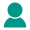•• ARTICLE
• STRING
• CONVERTER
• ENCRYPT
• NETWORK
• MORE
• ARTICLE
STRING
CONVERTER
ENCRYPT
NETWORK
MOREOnline Tools

# RSA Encryption and Decryption Online

If you need to know about RSA encryption algorithm, please carefully read the instructions of this tool to set relevant parameters correctly.
RSA Encryption and Decryption Online-summary

Online asymmetric RSA encryption and decryption tool supports public key encryption and private key decryption. Private key encryption, public key decryption. It supports the input of public and private keys in PEM and HEX formats. The input data and output results support String, Hex and Base64.

RSA Encryption and Decryption Online-instructions

Asymmetric encryption is a widely used data encryption algorithm, which uses different keys for encryption and decryption. RSA encryption algorithm is a widely used asymmetric encryption algorithm.
In RSA encryption algorithm, the key is divided into public key (public) and private key (private). Public and private keys are paired. For data encrypted with public key, only the corresponding private key can be decrypted. For data encrypted with private key, only the corresponding public key can be decrypted.

1. Mode:
ECB (Electronic Code Book) : This mode of operation is the simplest of all. In this mode, the whole data is divided into several segments, and then each segment is encrypted. Need to padding data for alignment.
3. Key Type : Select whether the input key is a public or private key.
4. Key Format :Select whether the input key is in pem format or hex format.